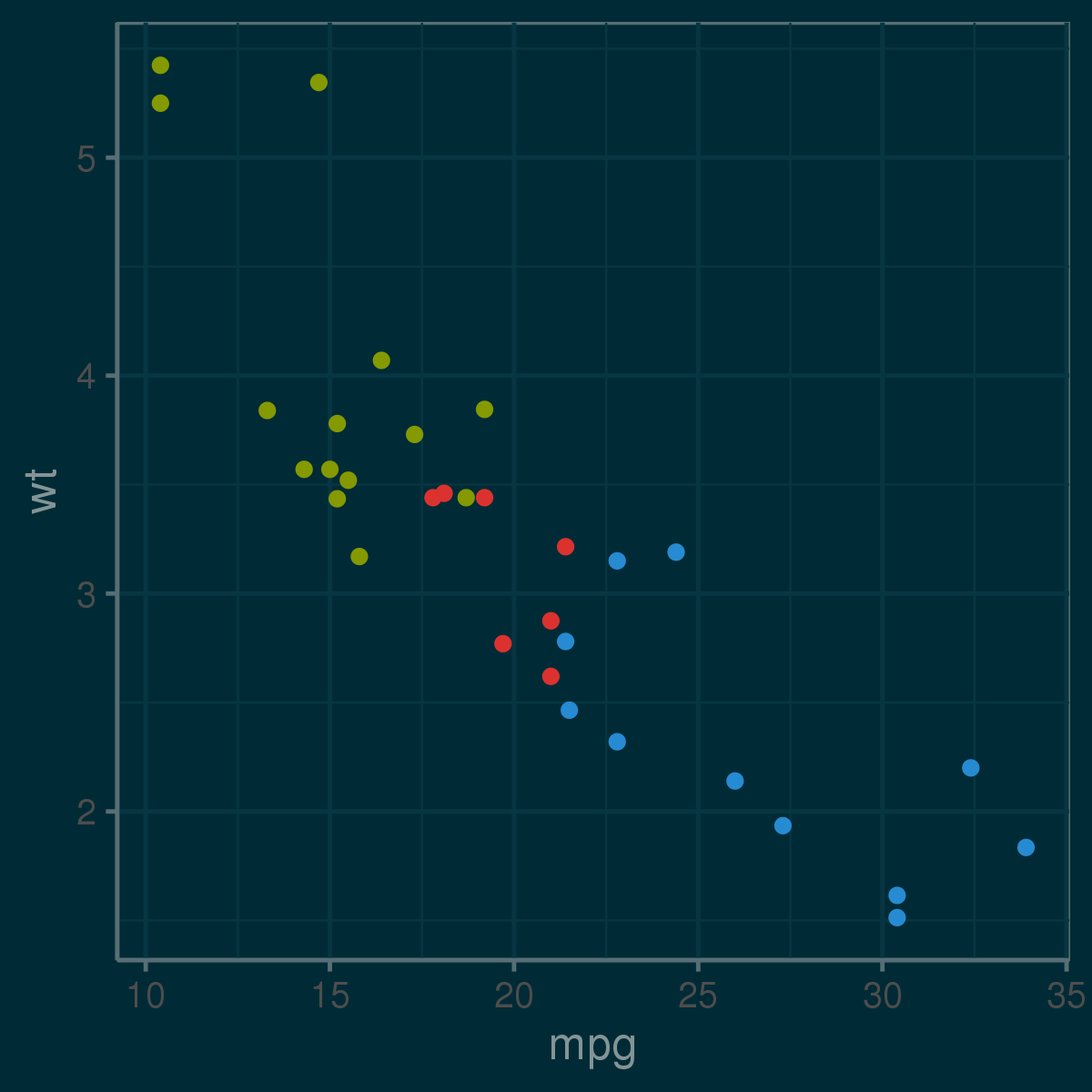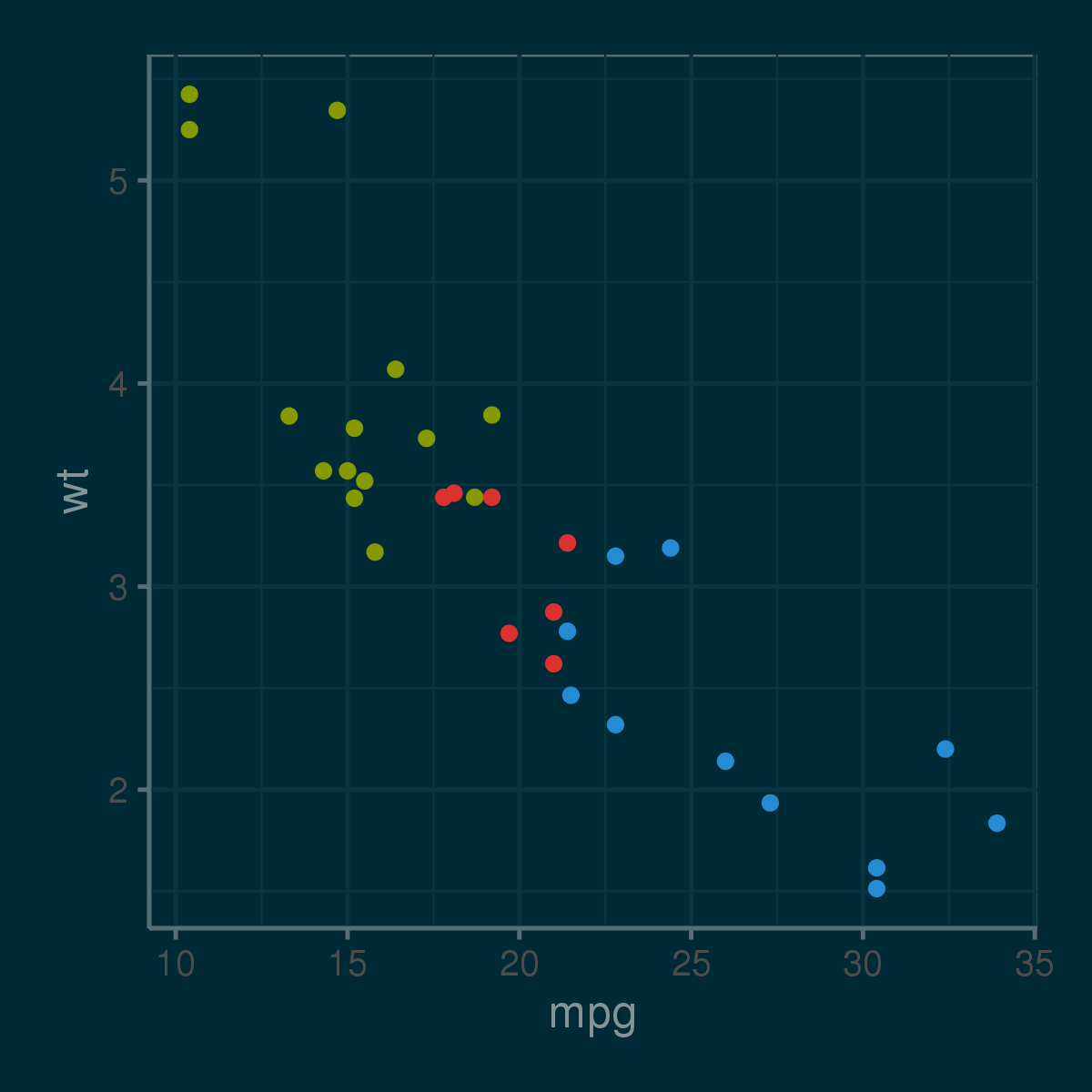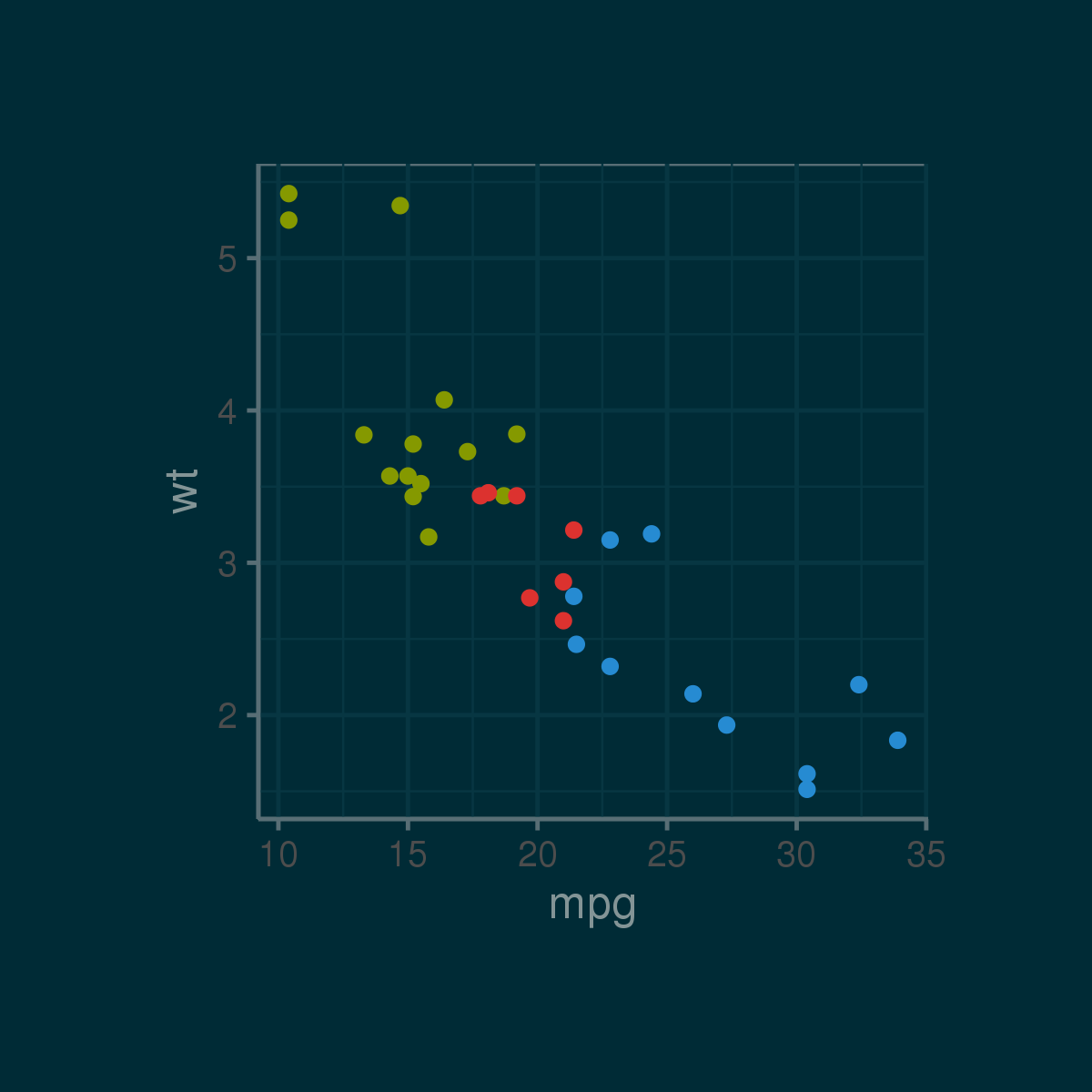## 1. 原图

```library(ggplot2)
library(ggthemes)
p <- ggplot(mtcars, aes(mpg, wt)) + geom_point(aes(colour=factor(cyl))) + guides(color=F)
p <- p + theme_solarized(light=FALSE) + scale_colour_solarized('blue')
ggsave("test0.png", units="in", dpi=300, width=4, height=4, device="png")```## 2. 第一次调整边界参数

```library(ggplot2)
library(ggthemes)
p <- ggplot(mtcars, aes(mpg, wt)) + geom_point(aes(colour=factor(cyl))) + guides(color=F)
p <- p + theme_solarized(light=FALSE) + scale_colour_solarized('blue')
p <- p + theme(plot.margin=unit(rep(1,4),'lines'))
ggsave("test1.png", units="in", dpi=300, width=4, height=4, device="png")```## 3. 第二次调整边界参数

```library(ggplot2)
library(ggthemes)
p <- ggplot(mtcars, aes(mpg, wt)) + geom_point(aes(colour=factor(cyl))) + guides(color=F)
p <- p + theme_solarized(light=FALSE) + scale_colour_solarized('blue')
p <- p + theme(plot.margin=unit(rep(3,4),'lines'))
ggsave("test2.png", units="in", dpi=300, width=4, height=4, device="png")```